JEE  >  Test: Class 12 Mathematics: CBSE Sample Question Paper- Term I (2021-22)

# Test: Class 12 Mathematics: CBSE Sample Question Paper- Term I (2021-22)

Test Description

## 50 Questions MCQ Test Sample Papers for Class 12 Medical and Non-Medical | Test: Class 12 Mathematics: CBSE Sample Question Paper- Term I (2021-22)

Test: Class 12 Mathematics: CBSE Sample Question Paper- Term I (2021-22) for JEE 2022 is part of Sample Papers for Class 12 Medical and Non-Medical preparation. The Test: Class 12 Mathematics: CBSE Sample Question Paper- Term I (2021-22) questions and answers have been prepared according to the JEE exam syllabus.The Test: Class 12 Mathematics: CBSE Sample Question Paper- Term I (2021-22) MCQs are made for JEE 2022 Exam. Find important definitions, questions, notes, meanings, examples, exercises, MCQs and online tests for Test: Class 12 Mathematics: CBSE Sample Question Paper- Term I (2021-22) below.
Solutions of Test: Class 12 Mathematics: CBSE Sample Question Paper- Term I (2021-22) questions in English are available as part of our Sample Papers for Class 12 Medical and Non-Medical for JEE & Test: Class 12 Mathematics: CBSE Sample Question Paper- Term I (2021-22) solutions in Hindi for Sample Papers for Class 12 Medical and Non-Medical course. Download more important topics, notes, lectures and mock test series for JEE Exam by signing up for free. Attempt Test: Class 12 Mathematics: CBSE Sample Question Paper- Term I (2021-22) | 50 questions in 40 minutes | Mock test for JEE preparation | Free important questions MCQ to study Sample Papers for Class 12 Medical and Non-Medical for JEE Exam | Download free PDF with solutions
 1 Crore+ students have signed up on EduRev. Have you?
Test: Class 12 Mathematics: CBSE Sample Question Paper- Term I (2021-22) - Question 1

### What is the principal value branch of cot–1x ?

Detailed Solution for Test: Class 12 Mathematics: CBSE Sample Question Paper- Term I (2021-22) - Question 1

The cotangent function is periodic so to calculate its inverse function we need to make the function bijective. For that we have to consider an interval in which all values of the function exist and do not repeat. For cotangent function this interval is considered as (0, π).

Thus when we take the inverse of the function the domain becomes range and the range becomes domain. Hence the principal value branch is the range of cot–1 x that is (0, π).

Test: Class 12 Mathematics: CBSE Sample Question Paper- Term I (2021-22) - Question 2

### What is the continuity of [x] at x = 3.5 ?

Detailed Solution for Test: Class 12 Mathematics: CBSE Sample Question Paper- Term I (2021-22) - Question 2

L.H. L=R.H. L = f(x)

Test: Class 12 Mathematics: CBSE Sample Question Paper- Term I (2021-22) - Question 3

### Choose the correct option for the given matrix.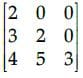Detailed Solution for Test: Class 12 Mathematics: CBSE Sample Question Paper- Term I (2021-22) - Question 3

A square matrix is called lower triangular if all the entries above the main diagonal are zero. Similarly, a square matrix is called upper triangular if all the entries below the main diagonal is zero.
Hence, given matrix is lower triangular matrix.

Test: Class 12 Mathematics: CBSE Sample Question Paper- Term I (2021-22) - Question 4

If a matrix has 4 rows and 3 columns then how many elements will be there in this matrix?

Detailed Solution for Test: Class 12 Mathematics: CBSE Sample Question Paper- Term I (2021-22) - Question 4

Matrix is represented by m × n
Where m = no. of rows & n = no. of column
And number of total elements = mn
If a matrix has 4 rows and 3 columns hen the number of elements in the matrix is 12.

Test: Class 12 Mathematics: CBSE Sample Question Paper- Term I (2021-22) - Question 5

What is the equation of the normal to the curve y = 3x2 – 7x + 5 at (0, 5)?

Detailed Solution for Test: Class 12 Mathematics: CBSE Sample Question Paper- Term I (2021-22) - Question 5

Tangent and normal are perpendicular to each other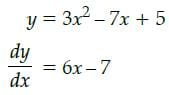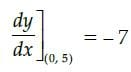∴ Slope of normal = 1/7
Equation of normal is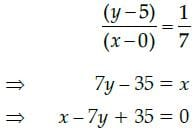Test: Class 12 Mathematics: CBSE Sample Question Paper- Term I (2021-22) - Question 6

If A is a square matrix of order 3, such that A(adj A) = 10I, then |adj A| is equal to

Detailed Solution for Test: Class 12 Mathematics: CBSE Sample Question Paper- Term I (2021-22) - Question 6

Consider the equation
Here, A(adj A) = 10 I
Then, |A| = 10
Where n is order of matrix
Here, = |A|3–1 = 102 = 100

Test: Class 12 Mathematics: CBSE Sample Question Paper- Term I (2021-22) - Question 7

R be the relation in the set N given by R = {(a, b) : a = b –2, b > 6}. Then, the correct option is:

Detailed Solution for Test: Class 12 Mathematics: CBSE Sample Question Paper- Term I (2021-22) - Question 7

Take b =8, ⇒ a = 6.
Hence, (6, 8) ∈ R

Test: Class 12 Mathematics: CBSE Sample Question Paper- Term I (2021-22) - Question 8

Evaluate the determinant of the matrix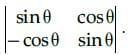Detailed Solution for Test: Class 12 Mathematics: CBSE Sample Question Paper- Term I (2021-22) - Question 8

The determinant of a square matrix of order 2 is given by the difference of the product of diagonal elements and the product of the offdiagonal elements.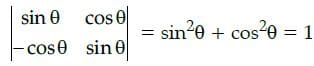Test: Class 12 Mathematics: CBSE Sample Question Paper- Term I (2021-22) - Question 9

Which of the function is not differentiable everywhere in R?

Detailed Solution for Test: Class 12 Mathematics: CBSE Sample Question Paper- Term I (2021-22) - Question 9

|x| is not differentiable at x=0

Test: Class 12 Mathematics: CBSE Sample Question Paper- Term I (2021-22) - Question 10

All the trigonometric functions have inverse functions irrespective of the domain.

Detailed Solution for Test: Class 12 Mathematics: CBSE Sample Question Paper- Term I (2021-22) - Question 10

For the inverse of a function to exist the function should be bijective which none of the trigonometric function is as they are periodic functions.

Test: Class 12 Mathematics: CBSE Sample Question Paper- Term I (2021-22) - Question 11

Let R be the relation in the set {5, 6, 7, 8} given by R = {(5, 6), (6, 6), (5, 5), (8, 8), (5, 7), (7, 7), (7, 6)}.

Detailed Solution for Test: Class 12 Mathematics: CBSE Sample Question Paper- Term I (2021-22) - Question 11

Let R be the relation in the set {1, 2,3, 4} is given by:
R = {(5,6), (6,6), (5,5), (8,8), (5,7), (7,7), (7,6)}
(a) (5,5), (6,6), (7,7), (8,8) ∈ R Therefore, R is reflexive.
(b) (5,6) ∈ R but (6,5) ∈ R. Therefore, R is not symmetric.
(c) If (5, 7) ∈ R and (7, 6) ∈ R then (5, 6) ∈ R. Therefore, R is transitive.

Test: Class 12 Mathematics: CBSE Sample Question Paper- Term I (2021-22) - Question 12

What is the equation of the tangent to the curve y – 5x2 = 0 and parallel to line 5x + y + 1 = 0 ?

Detailed Solution for Test: Class 12 Mathematics: CBSE Sample Question Paper- Term I (2021-22) - Question 12

Given equation of the curve is y – 5x2 = 0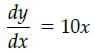Slope of the line 5x + y + 1 = 0 is – 5 slope of the tangent = slope of the parallel line
i.e., 10x = – 5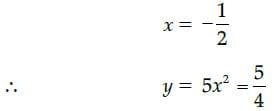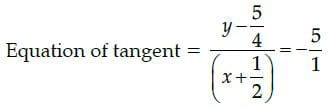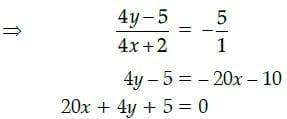Test: Class 12 Mathematics: CBSE Sample Question Paper- Term I (2021-22) - Question 13

if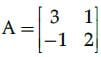then A2−5A + 7I2 is equal to :

Detailed Solution for Test: Class 12 Mathematics: CBSE Sample Question Paper- Term I (2021-22) - Question 13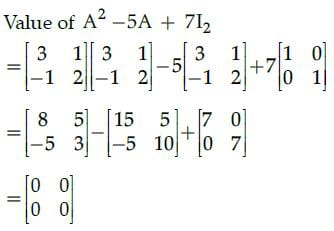Since, all the elements of matrix are zero. So, given matrix is null/zero matrix.

Test: Class 12 Mathematics: CBSE Sample Question Paper- Term I (2021-22) - Question 14

If y = log(cosex) then dy/dx will be:

Detailed Solution for Test: Class 12 Mathematics: CBSE Sample Question Paper- Term I (2021-22) - Question 14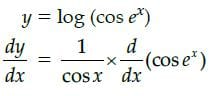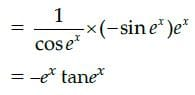Test: Class 12 Mathematics: CBSE Sample Question Paper- Term I (2021-22) - Question 15

For a square matrix A = [aij] the quantity calculated for any element aij in A as the determinant of thesquare sub-matrix of order (n – 1) obtained by leaving the ith row and jth column of A is known as:

Detailed Solution for Test: Class 12 Mathematics: CBSE Sample Question Paper- Term I (2021-22) - Question 15

The minor of an element aij in A is calculated as the determinant of the square sub-matrix of order (n-1) obtained by leaving the ith row and jth column of A.

Test: Class 12 Mathematics: CBSE Sample Question Paper- Term I (2021-22) - Question 16

Which of the following is satisfied by the given function y = sin10x−cos10x ?

Detailed Solution for Test: Class 12 Mathematics: CBSE Sample Question Paper- Term I (2021-22) - Question 16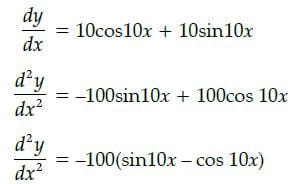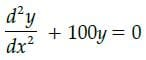Test: Class 12 Mathematics: CBSE Sample Question Paper- Term I (2021-22) - Question 17

If matrix A = [2 3 5], then the value of A.A' is:

Detailed Solution for Test: Class 12 Mathematics: CBSE Sample Question Paper- Term I (2021-22) - Question 17

A = [2 3 5]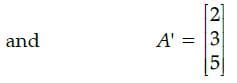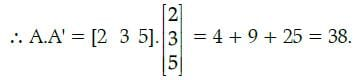Test: Class 12 Mathematics: CBSE Sample Question Paper- Term I (2021-22) - Question 18

The function y = 5x2 – 32x has a local minimum in the interval (0,10).

Detailed Solution for Test: Class 12 Mathematics: CBSE Sample Question Paper- Term I (2021-22) - Question 18

let f(x) = 5x2 – 32x
f '(x) = 10x – 32
10x – 32 = 0
f"(x) for x = 3.2 and f'(x)<0 for x >3.2 .

Test: Class 12 Mathematics: CBSE Sample Question Paper- Term I (2021-22) - Question 19

Z = 30x1 + 30x2, subject to x1 ≥ 0, x2 ≥ 0, x1 + 3x2 ≤ 6, 4x1 + 8x2 ≥ 16, x1 + x2 ≤ 4. The minimum value of Z occurs at

Detailed Solution for Test: Class 12 Mathematics: CBSE Sample Question Paper- Term I (2021-22) - Question 19

Corner Points are (0, 2), (2, 1), (4, 0) and (3, 1).
The objective function for (x, y) is 30x1 + 30x2.
After putting the corner points in the objective function, we get the minimum value of Z.

Test: Class 12 Mathematics: CBSE Sample Question Paper- Term I (2021-22) - Question 20

A rectangle of maximum area is inscribed in a circle of radius R.Then which of the following is true?

Detailed Solution for Test: Class 12 Mathematics: CBSE Sample Question Paper- Term I (2021-22) - Question 20

On applying Second Derivative test,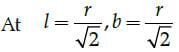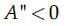Test: Class 12 Mathematics: CBSE Sample Question Paper- Term I (2021-22) - Question 21

Let T be the set of all triangles in a plane with R is a relation in T given by R = {(T1, T2) : T1 is congruent to T2}. Then R is

Detailed Solution for Test: Class 12 Mathematics: CBSE Sample Question Paper- Term I (2021-22) - Question 21

For reflexive, T1 is congruent to T1
⇒ (T1,T1) Î R For symmetric, (T1,T2) ∈ R ⇒ T1 is congruent to T2 ⇒ T2 is congruent to T1 ⇒ (T2,T1) ∈ R. Hence it is symmetric.
For transitive, (T1, T2) ∈ R ⇒ T1 is congruent to T2 and (T2,T3) ∈ R ⇒ T2 is congruent to T3 which implies T1 is congruent to T3 ⇒ (T1,T3) ∈ R. Hence, it is transitive.
Hence, R is an equivalence relation.

Test: Class 12 Mathematics: CBSE Sample Question Paper- Term I (2021-22) - Question 22

Consider the function 3x4 +20x3 −36x2 +44 in the interval [−5,10] , function is maximum at

Detailed Solution for Test: Class 12 Mathematics: CBSE Sample Question Paper- Term I (2021-22) - Question 22

Let f(x) = 3x4 + 20x3 – 36x2 + 44
f '(x) = 12x3 + 60x2 – 72x
f'(x) = 0
12x3 + 60x2 – 72x = 0
12x (x2 + 5x – 6) = 0
12x (x – 3) (x – 2) = 0
x = 0, 2, 3
f''(x) = 36x2 + 120x – 72
f''(0) = –72 < 0
f''(2) = 312 > 0
f''(3) = 612 > 0
∴ function has a maxima at x = 0

Test: Class 12 Mathematics: CBSE Sample Question Paper- Term I (2021-22) - Question 23

The graph of x ≤ 2 and y ≥ 2 will be situated in quadrants.

Detailed Solution for Test: Class 12 Mathematics: CBSE Sample Question Paper- Term I (2021-22) - Question 23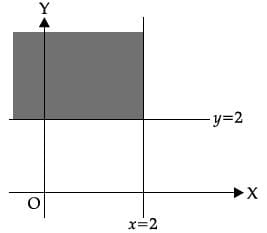Test: Class 12 Mathematics: CBSE Sample Question Paper- Term I (2021-22) - Question 24

What is the value of l in the function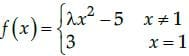provided function is continuous at x = 1?

Detailed Solution for Test: Class 12 Mathematics: CBSE Sample Question Paper- Term I (2021-22) - Question 24

For a function to be continuous L.H. L=R.H. L= f (x)

Test: Class 12 Mathematics: CBSE Sample Question Paper- Term I (2021-22) - Question 25

If A is a 3 × 3 matrix such that |A| = 8, then |3A| equals

Detailed Solution for Test: Class 12 Mathematics: CBSE Sample Question Paper- Term I (2021-22) - Question 25

Here |A| = 8
Then |3A| = 33|A| = 27 × 8 = 216

Test: Class 12 Mathematics: CBSE Sample Question Paper- Term I (2021-22) - Question 26

The function f(x) = tan x – x

Detailed Solution for Test: Class 12 Mathematics: CBSE Sample Question Paper- Term I (2021-22) - Question 26

We have
f(x) = tan x – x
On differentiating with respect to x, we
get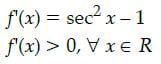So, f(x) always increases.

Test: Class 12 Mathematics: CBSE Sample Question Paper- Term I (2021-22) - Question 27

What is the domain of the cot–1 x ?

Detailed Solution for Test: Class 12 Mathematics: CBSE Sample Question Paper- Term I (2021-22) - Question 27

The cot function is periodic so to calculate its inverse function we need to make the function bijective. For that we have to consider an interval in which all values of the function exist and do not repeat. Now for the inverse of a function the domain becomes range and the range becomes domain. Thus the range of cot function, that is, (-∞, ∞) becomes the domain of inverse function.

Test: Class 12 Mathematics: CBSE Sample Question Paper- Term I (2021-22) - Question 28

If A is skew symmetric matrix of order 3, then the value of |A| is

Detailed Solution for Test: Class 12 Mathematics: CBSE Sample Question Paper- Term I (2021-22) - Question 28

Determinant value of skew symmetric matrix is always '0'.

Test: Class 12 Mathematics: CBSE Sample Question Paper- Term I (2021-22) - Question 29

The function f(x) = 4sin3x – 6 sin2x + 12 sinx + 100 is strictly

Detailed Solution for Test: Class 12 Mathematics: CBSE Sample Question Paper- Term I (2021-22) - Question 29

Given that, f(x) = 4sin3x – 6 sin2x + 12 sinx 100
On differentiating with respect to x, we get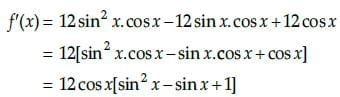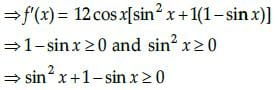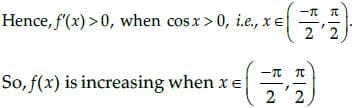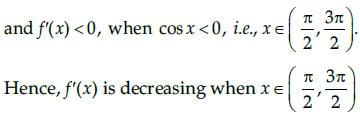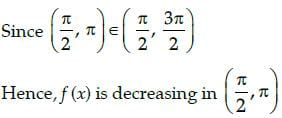Test: Class 12 Mathematics: CBSE Sample Question Paper- Term I (2021-22) - Question 30

If A = {1, 2}, B = {3,4, 5} and f = {(1, 3), (2, 5)} is a function from A to B, then f(x) is

Detailed Solution for Test: Class 12 Mathematics: CBSE Sample Question Paper- Term I (2021-22) - Question 30

Given, A = {1, 2}, B = {3,4, 5} and f : A → B is defined as f = {(1, 3), (2, 5)} i.e., f(1) = 3, f(2) = 5.
We can see that the images of distinct elements of A under f are distinct. So, f is one-one.

Test: Class 12 Mathematics: CBSE Sample Question Paper- Term I (2021-22) - Question 31

The function f(x) = e|x| is

Detailed Solution for Test: Class 12 Mathematics: CBSE Sample Question Paper- Term I (2021-22) - Question 31

Given that,
f (x) = e|x|

The functions ex and |x| are continuous functions for all real value of x. Since ex is differentiable everywhere but |x| is non-differentiable at x = 0.
Thus, the given functions f(x) = e|x| is continuous everywhere but not differentiable at x = 0.

Test: Class 12 Mathematics: CBSE Sample Question Paper- Term I (2021-22) - Question 32

The matrix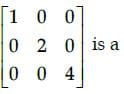Detailed Solution for Test: Class 12 Mathematics: CBSE Sample Question Paper- Term I (2021-22) - Question 32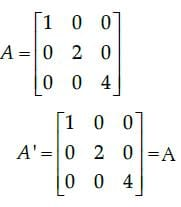So, the given matrix is a symmetric matrix. [Since, in a square matrix A, if A’ = A, then A is called symmetric matrix.]

Test: Class 12 Mathematics: CBSE Sample Question Paper- Term I (2021-22) - Question 33

Z = 7x + y, subject to 5x + y £ 5, x + y ³ 3, x ³ 0, y ≥ 0. Maximum value of Z occurs at

Detailed Solution for Test: Class 12 Mathematics: CBSE Sample Question Paper- Term I (2021-22) - Question 33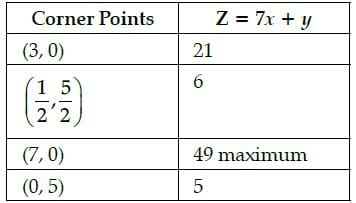Test: Class 12 Mathematics: CBSE Sample Question Paper- Term I (2021-22) - Question 34

Which of the following functions is decreasing on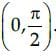Detailed Solution for Test: Class 12 Mathematics: CBSE Sample Question Paper- Term I (2021-22) - Question 34

In the given interval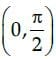f(x) = cos x
On differentiating with respect to x, we get f´(x) = – sin x
which gives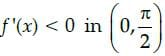Hence, f(x) = cosx is decreasing in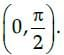Test: Class 12 Mathematics: CBSE Sample Question Paper- Term I (2021-22) - Question 35

If A and B are symmetric matrices of same order, then AB – BA is a:

Detailed Solution for Test: Class 12 Mathematics: CBSE Sample Question Paper- Term I (2021-22) - Question 35

A and B are symmetric matrices.
⇒ A = A’ and B = B’
Now, (AB – BA)’ = (AB)’ – (BA)’ ...(i)
⇒ (AB – BA)’=B’A’ – A’B’ [By reversal law]
⇒ (AB – BA)’ = BA – AB [From Eq. (i)]
⇒ (AB – BA)’ = –(AB – BA)
⇒ (AB – BA) is a skew-symmetric matrix.

Test: Class 12 Mathematics: CBSE Sample Question Paper- Term I (2021-22) - Question 36

The principal value of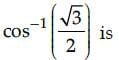Detailed Solution for Test: Class 12 Mathematics: CBSE Sample Question Paper- Term I (2021-22) - Question 36

The principal value of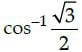means that we need to find an angle in the principal branch of the function where the cosine function is equal to √3/2 . Hence the required value
is π/6

Test: Class 12 Mathematics: CBSE Sample Question Paper- Term I (2021-22) - Question 37

The domain of sin–1 x is :

Detailed Solution for Test: Class 12 Mathematics: CBSE Sample Question Paper- Term I (2021-22) - Question 37

The domain of sin-1 x is [-1, 1].

Test: Class 12 Mathematics: CBSE Sample Question Paper- Term I (2021-22) - Question 38

if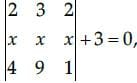then the value of x is

Detailed Solution for Test: Class 12 Mathematics: CBSE Sample Question Paper- Term I (2021-22) - Question 38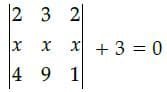On expanding along R1
2(x – 9x) – 3(x – 4x)+ 2(9x – 4x) + 3 = 0
2(–8x) – 3(–3x) + 2(5x) + 3 = 0
– 16x + 9x + 10x + 3 = 0
3x + 3 = 0
3x = –3
x = - 3/3
x = –1

Test: Class 12 Mathematics: CBSE Sample Question Paper- Term I (2021-22) - Question 39

The contentment obtained after eating x units of a new dish at a trial function is given by the functionf(x) = x3 + 6x2 + 5x + 3. The marginal contentment when 3 units of dish are consumed is ____________.

Detailed Solution for Test: Class 12 Mathematics: CBSE Sample Question Paper- Term I (2021-22) - Question 39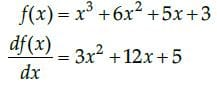At x = 3,
Marginal contentment
= 3x (3)2 + 12 x 3 + 5
= 27+ 36 +5
68 units.

Test: Class 12 Mathematics: CBSE Sample Question Paper- Term I (2021-22) - Question 40

The matrix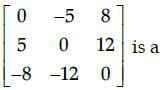Detailed Solution for Test: Class 12 Mathematics: CBSE Sample Question Paper- Term I (2021-22) - Question 40

We know that, in a square matrix, if bij = 0 when i ≠ j then it is said to be a diagonal matrix. Here, b12, b13…. ≠ 0
so the given matrix is not a diagonal matrix.
Now,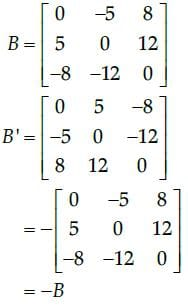So, the given matrix is a skew-symmetric matrix, since we know that in a square matrix B, if B’ = − B, then it is called skew-symmetric matrix.

Test: Class 12 Mathematics: CBSE Sample Question Paper- Term I (2021-22) - Question 41

A dealer in rural area wishes to purchase a number of sewing machines. He has only ₹5,760 to invest and
has space for at most 20 items for storage. An electronic sewing machine cost him ₹360 and a manually
operated sewing machine ₹240. He can sell an electronic sewing machine at a profit of ₹22 and a manually
operated machine at a profit of ₹18. Assume that the electronic sewing machines he can sell is x and that
of manually operated machines is y.The objective function is _______.

Test: Class 12 Mathematics: CBSE Sample Question Paper- Term I (2021-22) - Question 42

The maximum value of sin x. cos x is

Detailed Solution for Test: Class 12 Mathematics: CBSE Sample Question Paper- Term I (2021-22) - Question 42

Let us assume that,
f(x) = sin x cos x
Now, we know that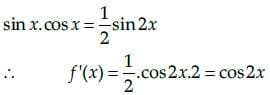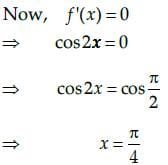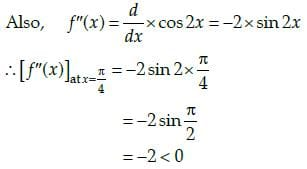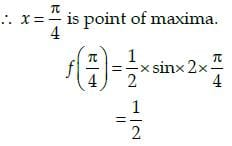Test: Class 12 Mathematics: CBSE Sample Question Paper- Term I (2021-22) - Question 43

The maximum value of (1/x)is:

Detailed Solution for Test: Class 12 Mathematics: CBSE Sample Question Paper- Term I (2021-22) - Question 43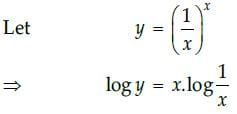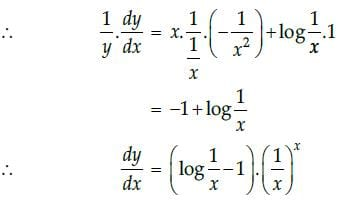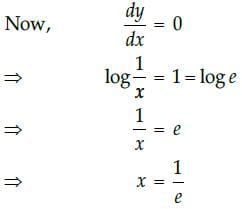Hence, the maximum value of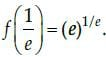Test: Class 12 Mathematics: CBSE Sample Question Paper- Term I (2021-22) - Question 44

From the figure, which point is not belongs to feasible region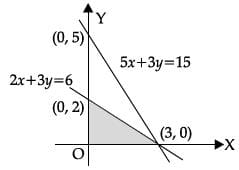Test: Class 12 Mathematics: CBSE Sample Question Paper- Term I (2021-22) - Question 45

Which of the given values of x and y make the following pair of matrices equal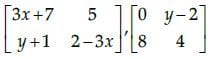Detailed Solution for Test: Class 12 Mathematics: CBSE Sample Question Paper- Term I (2021-22) - Question 45

It is given that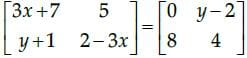Equating the corresponding elements, we get
3x + 7 = 0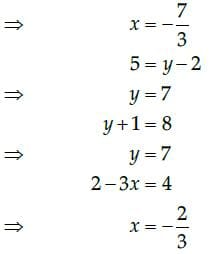We find that on comparing the corresponding elements of the two matrices, we get two different values of x, which is not possible.
Hence, it is not possible to find the values of x and y for which the given matrices are equal.

Test: Class 12 Mathematics: CBSE Sample Question Paper- Term I (2021-22) - Question 46

Case - Study

The Relation between the height of the plant (y in cm) with respect to exposure to sunlight is governed
by the following equation y = 4x -1/2x2 . where x is the number of days exposed to sunlight.The rate of growth of the plant with respect to sunlight is ______ .

Detailed Solution for Test: Class 12 Mathematics: CBSE Sample Question Paper- Term I (2021-22) - Question 46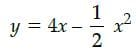∴ rate of growth of the plant with respect
to sunlightTest: Class 12 Mathematics: CBSE Sample Question Paper- Term I (2021-22) - Question 47

Case - Study

The Relation between the height of the plant (y in cm) with respect to exposure to sunlight is governed
by the following equation y = 4x -1/2x2 . where x is the number of days exposed to sunlight.What is the number of days it will take for the plant to grow to the maximum height?

Detailed Solution for Test: Class 12 Mathematics: CBSE Sample Question Paper- Term I (2021-22) - Question 47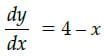The number of days it will take for the plant to grow to the maximum height,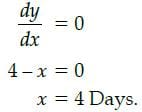Test: Class 12 Mathematics: CBSE Sample Question Paper- Term I (2021-22) - Question 48

Case - Study

The Relation between the height of the plant (y in cm) with respect to exposure to sunlight is governed
by the following equation y = 4x -1/2x2 . where x is the number of days exposed to sunlight.What is the maximum height of the plant?

Detailed Solution for Test: Class 12 Mathematics: CBSE Sample Question Paper- Term I (2021-22) - Question 48

We have, number of days for maximum height of plant = 4 Days
∴ Maximum height of plant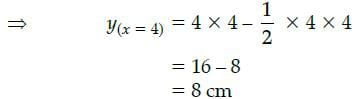Test: Class 12 Mathematics: CBSE Sample Question Paper- Term I (2021-22) - Question 49

Case - Study

The Relation between the height of the plant (y in cm) with respect to exposure to sunlight is governed
by the following equation y = 4x -1/2x2 . where x is the number of days exposed to sunlight.What will be the height of the plant after 2 days?

Detailed Solution for Test: Class 12 Mathematics: CBSE Sample Question Paper- Term I (2021-22) - Question 49

Height of plant after 2 days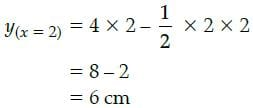Test: Class 12 Mathematics: CBSE Sample Question Paper- Term I (2021-22) - Question 50

Case - Study

The Relation between the height of the plant (y in cm) with respect to exposure to sunlight is governed
by the following equation y = 4x -1/2x2 . where x is the number of days exposed to sunlight.If the height of the plant is 7/2 cm, the number of days it has been exposed to the sunlight is _____ .

Detailed Solution for Test: Class 12 Mathematics: CBSE Sample Question Paper- Term I (2021-22) - Question 50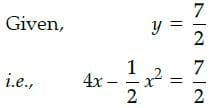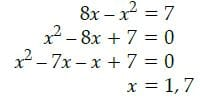We will take x = 1, because it will take 4 days for the plant to grow to the maximum height i.e. 8 cm and 7/2 cm is not maximum height so, it will take less than 4 days. i.e., 1 Day.

## Sample Papers for Class 12 Medical and Non-Medical

103 docs|4 tests
 Use Code STAYHOME200 and get INR 200 additional OFF Use Coupon Code
Information about Test: Class 12 Mathematics: CBSE Sample Question Paper- Term I (2021-22) Page
In this test you can find the Exam questions for Test: Class 12 Mathematics: CBSE Sample Question Paper- Term I (2021-22) solved & explained in the simplest way possible. Besides giving Questions and answers for Test: Class 12 Mathematics: CBSE Sample Question Paper- Term I (2021-22), EduRev gives you an ample number of Online tests for practice

103 docs|4 tests